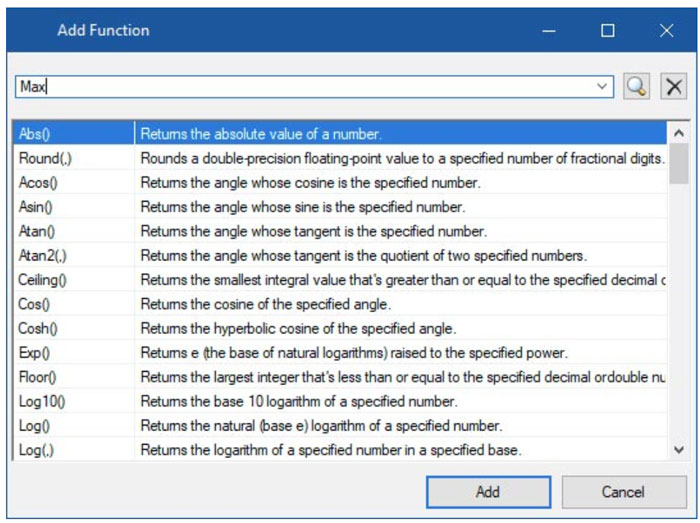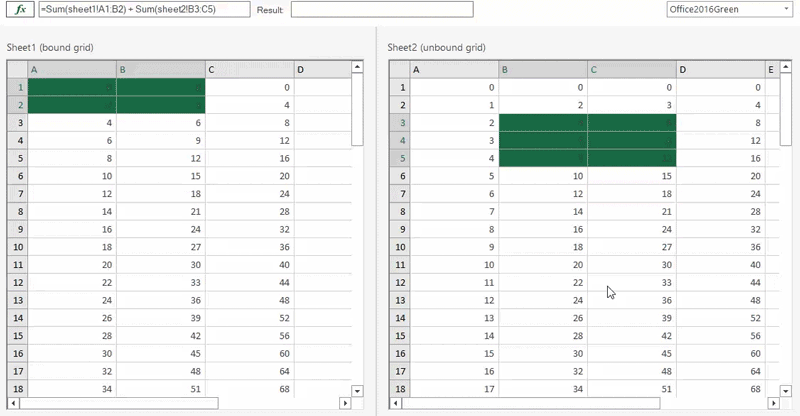﻿ Introducing CalcEngine for .NET | ComponentOne# Introducing CalcEngine for .NET

The ComponentOne CalcEngine is a .NET Standard library that can be used on any platform for runtime parsing and evaluation of expressions.

This library is useful for:

• Parsing and evaluation of expressions (like Microsoft Excel).
• Calculating the sales projections or statistical calculations to find patterns in data.
• Evaluating algebraic expressions, mathematical functions, and formulas using variables.

CalcEngine is available for all .NET platforms within ComponentOne, and the library is available as NuGet package on nuget.org. You can get the samples by installing WinForms Edition.

In this article, we will walk through the major features of CalcEngine and show how they work.

### Get Started Using CalcEngine

To start using CalcEngine, create a .NET Framework 4.6.2+ or a .NET Core application and add the C1.CalcEngine NuGet package to the project from nuget.org.

#### Creating Simple Expressions

Creating & evaluating expressions with CalcEngine is straight forward and familiar if you’ve ever used Excel. H ere we show how to create a simple expression assigned to the CalcEngine’s Expression property and how to use the Evaluate function to get the result.

``````var calcEngine = new C1CalcEngine();
calcEngine.Expression = “5+2”;
var result = calcEngine.Evaluate()
``````

CalcEngine also supports more complex expressions with functions. It supports text, datetime, math, logical, conversion & aggregate functions from Excel apart from various constants and operators.For a complete list of supported functions, please refer to the documentation. If you want to add a custom function to the library you can do that using the AddFunction method.

#### Perform Calculations Across a Data Source with Data Binding

CalcEngine can be bound to data sources like lists, arrays, or a data table. This helps in performing calculations over the collection of objects and data fields. Here we have a collection of Store objects and use CalcEngine to apply expressions on the entire collection or a single object.

``````public class Store
{
public int StoreId { get; set; }
public double Sales { get; set; }
}

var collection = new List<Store>() { new Store { StoreId = 27, Sales = 150000.00 }, new Store { StoreId = 15, Sales = 20000.00 } };

// 1\. When you have to apply expression over an object:
var engine = new C1CalcEngine ();
engine.DataSource = collection;
engine.Expression = "[Sales]*2";
``````
``````// 2\. When you have collection and aggregate function:
var engine = new C1CalcEngine ();
engine.DataSource = collection;
engine.Expression = "Sum([Sales])";
var result = engine.Evaluate();
``````
``````// 3\. When you have collection and need evaluate one entry:
var engine = new C1CalcEngine();
engine.DataSource = collection;
engine.CurrentIndex = 1;
engine.Expression = "[Sales] / Sum([Sales])";
var result = engine.Evaluate();
``````

#### Working with Multiple Data Collections

When the information is spread across several collections, it becomes difficult to work with such data together. This is where cross-referencing can be helpful. The built-in Excel engine’s cross-sheet referencing can be used in such cases. In cross-sheet references, a cell reference includes a reference to a cell in another sheet.

Like Excel, it can be used in a formula wherein the name of the target worksheet is entered followed by an exclamation (!) before the cell reference or cell range reference.

`calcEngine.Expression = "=Sum(Sheet1!A3:B7) + Sum(Sheet2!A3:B7)";`

To be able to create expressions such as above each data source should derive from IDataSheet implementing the GetValue() function:

`````` public class SheetTable : DataTable, IDataSheet
{
public string Name
{
get => TableName;
set => TableName = value;
}

public object GetValue(int col, int row)
{
return Rows[row][col];
}
}

``````

Here, the GetValue returns a value from a particular row or a column.

For the purpose of this article we demonstrate a simple data source that uses the SheetTable class implemented above:

``````//Method to generate data for C1CalcEngine
private const string columnNames = "ABCDEF";

var sheet1 = GetDataTable("Sheet1");
var sheet2 = GetDataTable("Sheet2");

public static SheetTable GetDataTable(string sheetName)
{
var table = new SheetTable();
table.Name = sheetName;
foreach (var col in columnNames)
for (int i = 0; i < 100; i++)
table.Rows.Add(new object[] { i * 2, i * 3, i * 4, i * 5, i * 6, i * 7 });
return table;
}
``````

Finally, cross-sheet expressions can be created as below:

`````` C1CalcEngine calcEngine = new C1CalcEngine();

calcEngine.DataSource = new List<IDataSheet> { sheet1, sheet2 };

//Assign the cross sheet reference expression to be calculated by C1CalcEngine
calcEngine.Expression = "=Sum(Sheet1!A3:B7) + Sum(Sheet2!A3:B7)";

//Invoke the TryEvaluate method of C1CalcEngine to calculate the expression
var res = calcEngine.TryEvaluate(out object result) ? result.ToString() : calcEngine.GetErrors().FirstOrDefault()?.FullMessage ?? "";
``````

An example of cross-sheet referencing is shown in the below image where expressions are parsed from cell references.

This sample is available with ComponentOne WinForms Edition: